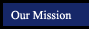Geometry Postulates and Theorems
textbook tutorials - definitions - constructions - postulates and theorems - internet activities and resources
 symmetry and similarity points, lines and planes parallel lines triangles quadrilaterals polygons circles 3-Dimensional Geometry
Related Thorems Polygon Theorems
The angles that form a linear pair are supplementary. In a polygon with n sides the sum of the angle measures is (n - 2) 180.
Vertical angles are congruent. Exterior Angles of a Regular Polygon
The sum of the measures of the exterior angles of any polygon is 360.
Two lines are perpendicular if and only if